In :
import pandas as pd
import numpy as np
import matplotlib.pyplot as plt
pd.set_option('display.mpl_style', 'default')
%matplotlib inline

In :
df = pd.read_csv('GPA.txt')


# The GPAs¶

In :
df['GPA'].value_counts()

Out:
4.000000    1708
0.000000     221
3.000000      19
1.000000      17
2.000000      17
3.333333       5
3.500000       5
3.800000       5
2.137266       4
0.768703       4
2.039216       3
3.446780       3
3.400000       3
3.666667       3
2.666667       3
...
3.966339    1
3.163170    1
3.379522    1
3.264674    1
2.989474    1
3.141395    1
3.476923    1
2.501581    1
3.950600    1
0.955029    1
3.639726    1
0.146689    1
2.687596    1
2.995737    1
3.673895    1
Length: 8153, dtype: int64

This tells us something about the underlying algorithm: 4.0 is by far the most common score, so this algorithm doesn't output a smooth score. The most common scores are the round numbers: 0.0, 1.0, 2.0, and 3.0. Interesting. (weird?)

I'd be interested to know what's going on here.

Before I start, how much data do we have available? Let's see!

# Total data points, by author count¶

In :
totals_by_author_count = df.groupby('AuthorCount').aggregate(lambda x: len(x))
totals_by_author_count.plot(kind='bar', figsize=(13, 3))

Out:
<matplotlib.axes.AxesSubplot at 0x517e7d0>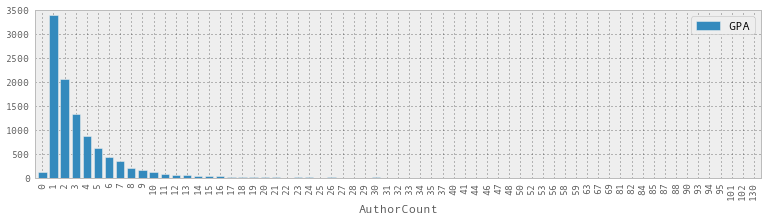So let's just look at author counts up to 10, say. (also, what's an author count of 0? Interesting.)

There are a ton of 4s, so this is obviously a score that this GPA algorithm thinks is important. Notice that as we go down, the percentage of 4s allocated decreases dramatically.

# Percentage of 4s, by author count¶

In :
fours_by_author_count = df.groupby('AuthorCount').aggregate(lambda x: float(np.count_nonzero(x['GPA'] == 4)) / len(x))
fours_by_author_count[:11].plot(kind='bar', figsize=(13, 3))

Out:
<matplotlib.axes.AxesSubplot at 0x570ca90>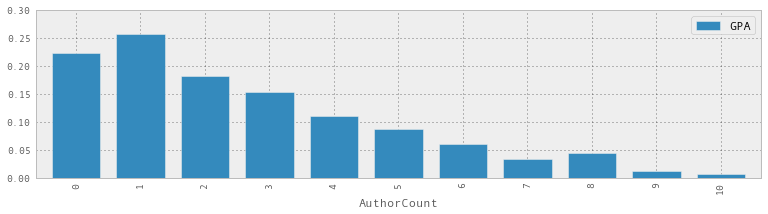Let's look at the median!

# Median score, by author count¶

In :
medians_by_author_count = df.groupby('AuthorCount').aggregate(lambda x: np.median(x['GPA']))
medians_by_author_count[:11].plot(kind='bar', figsize=(13, 3))

Out:
<matplotlib.axes.AxesSubplot at 0x5bb25d0>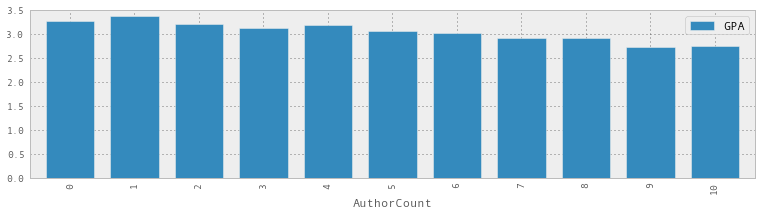This is pretty interesting. It's quite different from the "percentage of 4s" graph -- it's much much flatter. The highest median GPA is about 3.4, going down to 2.7 or so.

I wonder what happens if we find the median GPA, excluding all the 4s?

# Let's ignore GPAs of 4 entirely.¶

In :
medians_by_author_count_without_4 = df.groupby('AuthorCount').aggregate(lambda x: np.median(x['GPA'][x['GPA'] != 4]))
medians_by_author_count_without_4[:11].plot(kind='bar', figsize=(13, 3))

Out:
<matplotlib.axes.AxesSubplot at 0x5bd1510>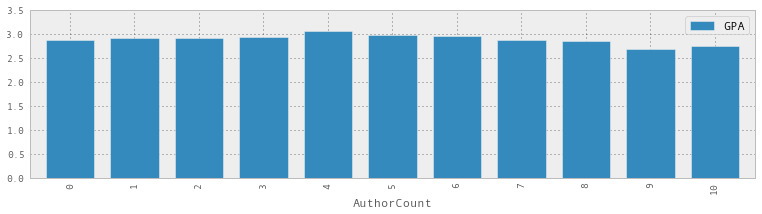This distribution is super different! It's hard to tell from the graph, but the maximum is actually at 4 authors, and then it goes down around 9 or 10. So it seems like the author count influences whether your GPA is 4 or not really strongly, but if your GPA is smaller than 4 it doesn't have as strong of an effect.

Let's look more closely at the GPA distributions for a few different author counts:

In :
def draw_hist(sub_df, ax=None, title=None):
gpa_col = sub_df['GPA']
ax.set_title(title)
gpa_col[gpa_col != 4].hist(bins=20, figsize= (20, 10), ax=ax)
_, axes = plt.subplots(3,2)
draw_hist(df[df.AuthorCount == 1], ax=axes, title="Author count 1")
draw_hist(df[df.AuthorCount == 2], ax=axes, title="Author count 2")
draw_hist(df[df.AuthorCount == 3], ax=axes, title="Author count 3")
draw_hist(df[df.AuthorCount == 4], ax=axes, title="Author count 4")
draw_hist(df[df.AuthorCount == 5], ax=axes, title="Author count 5")
draw_hist(df[df.AuthorCount == 6], ax=axes, title="Author count 6")
plt.tight_layout()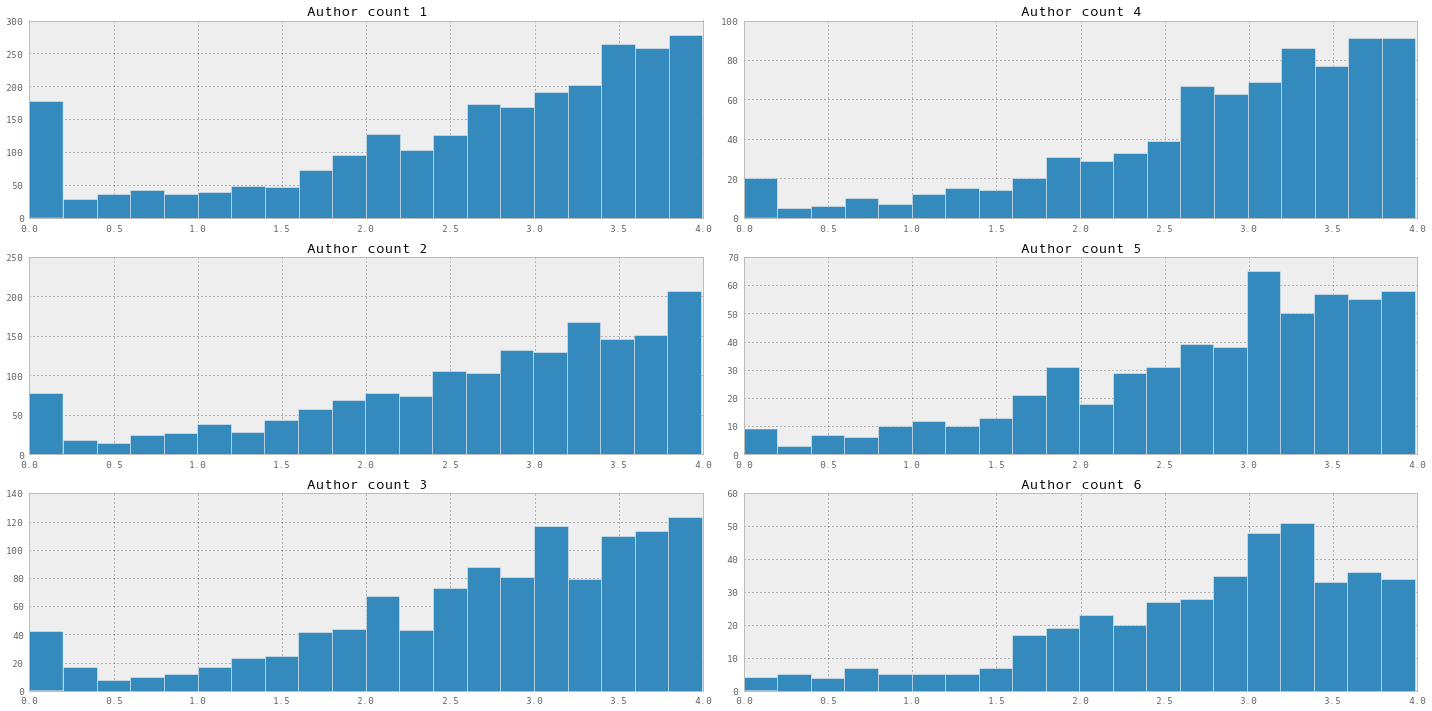Hmm. I can't draw any really good conclusions from this. Let's try plotting a few GPA percentiles, across numbers of authors:

In :
def get_percentile(p):
series = df.groupby('AuthorCount').aggregate(lambda x: np.percentile(x['GPA'][x['GPA'] != 4], p))[:11]
series.columns = [str(p)]
return series
ax = plt.axes()
get_percentile(90).plot(kind='line', figsize=(13, 3), ax=ax)
get_percentile(80).plot(kind='line', figsize=(13, 3), ax=ax)
get_percentile(70).plot(kind='line', figsize=(13, 3), ax=ax)
get_percentile(60).plot(kind='line', figsize=(13, 3), ax=ax)
get_percentile(50).plot(kind='line', figsize=(13, 3), ax=ax)

Out:
<matplotlib.axes.AxesSubplot at 0x69c7fd0>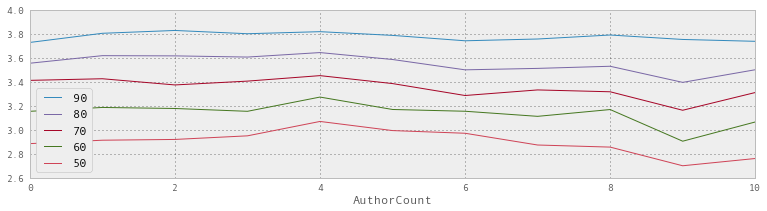These all look pretty flat to me! Certainly the highest median GPA is at 4, but the 90th percentile seems very very flat across different numbers of authors.